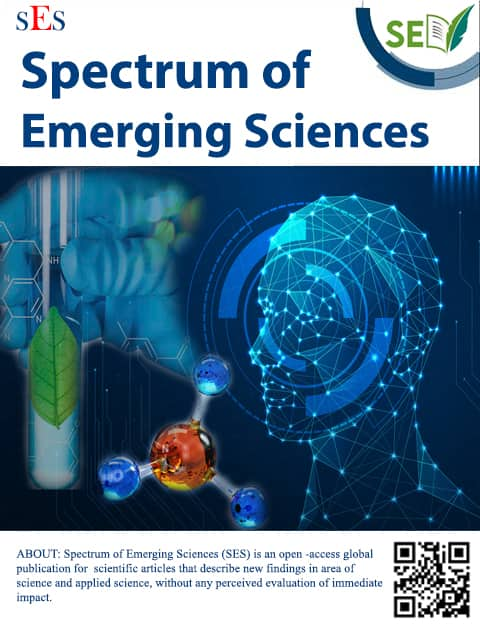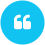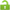ISSN:2583-2603

# Fractional PDE based Image Restoration using Grunwald Approximation

Author(s):

Email(s):

College of Engineering, Roorkee (COER), Roorkee, Uttarakhand 247667 IndiaB. D. Patel (2021)Fractional PDE based Image Restoration using Grunwald Approximation, Spectrum of Emerging Sciences, 1(1), pp. 16-20.View PDF

Please allow Pop-Up for this website to view PDF file.

 ARTICLE INFO ABSTRACT Original Research Article Received:   7 October 2021 Accepted: 24 October 2021     KEYWORDS PDE, Image de-noising, Fractional derivative, Euler-Lagrange equation, Grunwald approximation, This paper generalizes the computational applicability of non-integer ordered derivative based PDE (Partial Differential Equation) in image restoration process. The fractional derivative of Riemann-Liouville form gives the several choices of fractional order in image de-noising. The fractional form of Euler Lagrange equation is the non-integer ordered generalization of gradient type equations which can be used in minimization problems. The Grunwald approximation discretizes the fractional PDE used in image restoration, which shows some satisfactory results.

Introduction

The image processing using partial differential equation (PDE) has given a new direction in image restoration such as de-noising and enhancement [1-8]. Image restoration is often inevitable as a pre-processing of images especially for image segmentation and image compression. The computational applicability of appropriate numerical methodologies for the PDE models is a salient component of PDE-based image restoration. In image restoration, the integer ordered derivative is used for minimization of the total variation in the image. Many image restoration methodologies use the minimization of Lagrangian .

In this paper we have used the non-integer ordered derivative in image restoration. The non-integer order (fractional) derivative was theorized long back in the correspondence of Leibniz with L’Hospital in 1695 . The fractional derivative was developed as theoretical field of mathematics [11-14]. Research studies in scaling phenomena [15-17] and in classical mechanics [18-21] fractional calculus shows many interesting applications. The use of non-integer ordered derivative in diffusion process brings the dynamic nature of the non-linearity of the image filter. The use of fractional derivative in image restoration shows some encouraging results. The chaotic dynamics of the image processing can be optimized by the introduction of fractional Lagrangian to replace the non-integer order of the fractional derivative.

Section II of this paper gives the preliminaries idea of image restoration using Euler Lagrange (EL) equation. Fractional derivative and fractional EL equation have been discussed in section III, while Section IV deals with the concept of Grunwald approximation for discretization of fractional EL equation. In section V, the experimental results are shown and discussion is carried out. Finally, the conclusion is drawn in section VI.

II. Preliminaries

For a given image , the corrupted observed image, can be viewed as

,           (1)

where k is a blurring operator and e can be represented as the additive Gaussian noise. The de-noising of the image can be theorized as the minimization of a functional of gradient, represented as

, where           (2)

Here , can be defined as Lagrange constant and  represents the gradient operator defined as .  represents the distance of norm 2. If  p=1, the first term in is described as the total variation. The minimization problem (2) can be transformed into the differential form called the Euler-Lagrange (EL) equation, which is the minimization problem in 2D of the form

(3)

The EL equation states

(4)

where

i.e.               (5)

When the EL equation (4) is applied to equation (2), we get

(6)

Iterating the descent direction by the time step t, the resulting evolutionary EL equation can be represented as

(7)

where  is the constrained parameter.

III. De-noising Using Fractional Derivative

The fractional framework in partial differential equation has been used in a wide variety of computational problems like turbulence, chaotic dynamics, quantization etc. Riemann fractional derivative  is defined as

(8)

where equation is defined as  ordered left Riemann fractional derivative. Similarly, the  ordered right Riemann fractional derivative is defined as,

(9)

Here n is an integer such that  and  is the gamma function defined as ,

(10)

In (3), if we consider L = 0, the fractional derivative is called the Riemann-Liouville form, and for L =   it is the Liouville definition of fractional derivative [11,12]. Other definition of fractional derivative also exists. In this paper Riemann-Liouville form is considered without loss of generality. It is easy to see that these derivatives are non-local operator of convolution type.

The classical functional gradient  is defined as

(11)

which brings the essence of the x and y directional derivative. Now the fractional directional derivative operator can be viewed as , which can be defined as,

(12)

where .Now if we define the Lagrangian fractional functional as

(13)

then we can state the following lemma,

Lemma 1: Let L be an admissible Lagrangian function and , defined as (13), for , be the associated fractional functional and differentiable for all , then for all h, the differential is given by

, where .

Proof:

Since is differentiable for ,

for .                (14)

Again, Riemann-Liouville derivative is linear operator [11,12], which implies that,

(15)

As a consequence we obtain

(16)

Using Taylor expansion,

(17)

For two functions  we can state that ,

(18)

(19)

Using (17) and (19)

which proves the above Lemma.

Now let define the fractional divergence operator as , and we can state the following corollary,

Corollary: If the minimization of fractional 2D problem be

(20)

The fractional EL equation states that,

(21)

Proof: Using the Lemma 1 and classical Du Bois Reymond Lemma,  obtain the result (21).

Using the concept of fractional Lagrangian the denoising technique can be represented as

,       (22)

and when fractional Euler Lagrangian (EL) equation is applied to (22), we get

(23)

Iterating the descent direction by the time step t, we get,

(23)

where  is the constrained parameter.

Since the numerator  can not be zero in computation, the term can be regularized as

(24)

where  is very small.

IV. Numerical Approximation

The continuous domain representation of the fractional PDE requires discretization for computational feasibility. Right shifted Grunwald approximation can be used to discretize the equation (6) similar to finite difference approximation . The right shifted Grunwald formula can be represented as,

(25)

In image processing,  is bounded and , hence equation (25) can be approximated as

(26)

where  and are integers. Here , where  is the size of image. The two dimensional fractional PDE (6) used in processing of MRI can be discretized using equation as follows,

(27)

V. Results

For numerical experiments, we choose grey scale images. The image is perturbed with random valued additive Gaussian noise (zero mean and have a certain variance). The restoration procedure has been implemented Matlab R14. As shown in figure 1, we present the experimental results utilizing the gray-scale Zelda image in pixels. The original image is shown in  1(a) which is perturbed with Gaussian noise of zero mean and 0.01 variance, as shown in, figure 1(b). The perturbed image is de-noised by discretized fractional EL equation considering , and . In this example, the denoising is carried out for several choices of fractional order of the derivative after 20 iterations. The strong relationship between the PSNR of the denoised image and the fractional ordered  shows that there exists some maximum for which the PSNR of the denoised image is maximum in this process. In 1(c) the output image is for the fractional order , which is the denoised image derived from classical Euler Lagrange equation. For this image the PSNR is 21.13 dB. In 1(d) the image is denoised for the fractional order , for which the PSNR is 32.60 dB, which is maximum PSNR throughout the process, while the PSNR of the noisy image 1(b) is 11.96 dB.

(a)

(b)

(c)

(d)

Fig. 1. (a) Original Zelda image ( pixels) (b) Perturbed image with Gaussian noise of zero mean and 0.01 variance (c) restored image for fractiona order  and (d) restored image with fractional order

In figure 2, the curves show the relationship between the PSNR and the fractional order for different iterations (10 iterations and 20 iterations). The curve shows that there exists some point where the PSNR of the denoised image is maximum. When the fractional order is about 1, i.e. , the equation (23) can be viewed as diffusion process , while for , the same equation (23) can be defined as super diffusive process. For , the equation (23) follows the wave equation.

Fig. 2: The PSNR (dB) vs fractional order for different iterations

VI. Conclusion

The non-integer ordered partial differential equation (PDE) is used in this paper for the restoration of noisy image. The variation minimization problem is solved through the introduction of fractional derivative in classical Euler Lagrange equation. The non-integer ordered Euler Lagrange equation gives the scope of choices for several fractional ordered used in fractional derivative. The fractional variation is used to define the fractional generalization of gradient type equation for de-noising the image. The Grunwald approximation is used to discretize the fractional Euler-Lagrange equation and the numerical scheme is tested using different images which show some satisfactory results. The choice of fractional order affects the de-noising effect in the

## Recomonded Articles:

### Surgery to preserve the spleen with cardiac involvement in hydatid cyst diseases

Author(s): Parag Jain; Puneet Pal Singh

DOI: 10.55878/SES2021-1-1-1         Access:Open Access Read More

### Green Synthesis and Characterization of Zirconia Nanoparticles using Extract of Citrus sinensis Peels and its Comparative Antibacterial Activity with Cefotaxime

Author(s): Nelson Rai; Sambridhi Shah; Rajendra Joshi; Rajesh Pandit

DOI:         Access:Open Access Read More

### Image Fusion using Wavelet Transform: Systematic Literature Review

Author(s): Sushant Bindra; Mehak Piplani

DOI:         Access:Open Access Read More

### Effect of Plasticizer Concentration on the Physicochemical Properties of Starch-based Bioplastic Prepared from Potato Peels

Author(s): Binod Shrestha, Sambridhi Shah, Khagendra Chapain, Rajendra Joshi, Rajesh Pandit

DOI: 10.55878/SES2022-2-1-7         Access:Open Access Read More

### The Influence of Soap and Alcohol-based Cleanser on Human Skin

Author(s): Samrat Paudel; Rekina Shrestha; Pramod Poudel; Rameshwar Adhikari

DOI: 10.55878/SES2022-2-1-1         Access:Open Access Read More

### Carbon Quantum Dot and Application: A Review

Author(s): Bhupendra Kande, Prachi Parmar

DOI: 10.55878/SES2022-2-1-3         Access:Open Access Read More

### Thermal analysis of Exhaust manifold system using computational fluid dynamics

Author(s): Anand Pathak; Saurabh Deshmukh

DOI:         Access:Open Access Read More

### Stabilization and Solidification: A solution to the excessive pollution released by The INEOS Neville Plant

Author(s): Vania Munjar

DOI: 10.55878/SES2022-2-1-4         Access:Open Access Read More

### Employment of Green and Sustainable Protocols for C(sp3)-H bond Functionalization of 2-Methyl azaarenes and subsequent C-C bond formation

Author(s): Ratnakar Dutt Shukla; Byanju Rai

DOI:         Access:Open Access Read More

### Fractional PDE based Image Restoration using Grunwald Approximation

Author(s): B. D. Patel

DOI:         Access:Open Access Read More

### Sulphur and phosphorus containing compounds as green corrosion inhibitors

DOI:         Access:Open Access Read More

### Role of IoT, web services, and chemical science against coronavirus

Author(s): Reena Rawat

DOI:         Access:Open Access Read More

### Ramifications of Nanotechnology on Common Human Disorders

Author(s): Vania Munjar

DOI: 10.55878/SES2021-1-1-12         Access:Open Access Read More

### Study of etnano-medicinal plant of Himachal Pradesh

Author(s): Ruchi Sharma

DOI: 10.55878/SES2022-2-1-5         Access:Open Access Read More

### Green Synthesis of Zinc Oxide Nanomaterial

Author(s): Roli Jain

DOI: 10.55878/SES2022-2-1-6         Access:Open Access Read More

### Heavy Metal Tolerance and Toxicity Studies on- Indigenous Microflora and its Application for Bioremediation

Author(s): Shubhangi Jha, Pragya Kulkarni and Anamika Sharma

DOI: 10.55878/SES2022-2-2-3         Access:Open Access Read More

### PDE based Analysis for Propagation of Disturbance by Users Mobility in Mobile Network System

Author(s): Shathya Pranav Sujithra Rajesh Kannan

DOI: 10.55878/SES2022-2-2-1         Access:Open Access Read More

### Probabilistic Model for rGO-enriched nano-ceramics for electro-mechanical study

Author(s): Vania Munjar

DOI: 10.55878/SES2022-2-2-2         Access:Open Access Read More